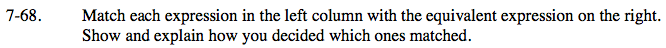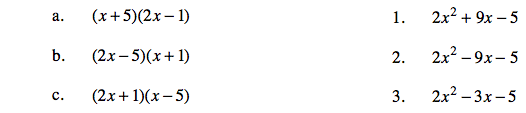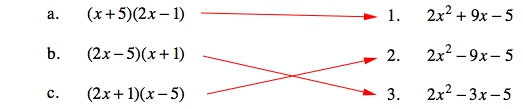### Home > AC > Chapter 7 > Lesson 7.2.2 > Problem7-68

7-68.

Match each expression in the left column with the equivalent expression on the right. Show and explain how you decided which ones matched. Homework Help ✎

 a. (x + 5)(2x − 1) 1. 2x2 + 9x − 5 b. (2x − 5)(x + 1) 2. 2x2 − 9x − 5 c. (2x + 1)(x − 5) 3. 2x2 − 3x − 5